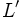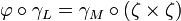# Homoclinism of Lie rings

## Definition

### Short definition

A homoclinism of Lie rings is a homologism of Lie rings with respect to the subvariety of the variety of Lie rings given by abelian Lie rings.

### Full definition

For any Lie ring$L$, let$Z(L)$ denote the center of$L$,$\operatorname{Inn}(L)$ denote the Lie ring of inner derivations of$L$ (explicitly, it is isomorphic to$L/Z(L)$, and$L'$ denote the derived subring of$L$.

Let$\gamma_L$ denote the mapping$\operatorname{Inn}(L) \times \operatorname{Inn}(L) \to L'$ that arises from the Lie bracket mapping$L \times L \to L'$, and then observing that this map is constant on the cosets of$Z(L) \times Z(L)$. Note that the mapping is$\mathbb{Z}$-bilinear.

A homoclinism of Lie rings$L$ and$M$ is a pair$(\zeta,\varphi)$ where$\zeta$ is a homomorphism of$\operatorname{Inn}(L)$ with <math\operatorname{Inn}(M)[/itex] and$\varphi$ is a homomorphism of$L'$ with$M'$, such that$\varphi \circ \gamma_L = \gamma_M \circ (\zeta \times \zeta)$.

## Related notions

Term Meaning
category of Lie rings with homoclinisms category whose objects are Lie rings and whose morphisms are homoclinisms
isoclinism of Lie rings an invertible homoclinism, or equivalently, a homoclinism where both the component homomorphisms are isomorphisms
homoclinism of groups the analogous concept for groups
homologism of Lie rings a more general concept of which homoclinisms are a special case. Homoclinisms are homologisms with respect to the subvarety of abelian groups in the variety of Lie rings.